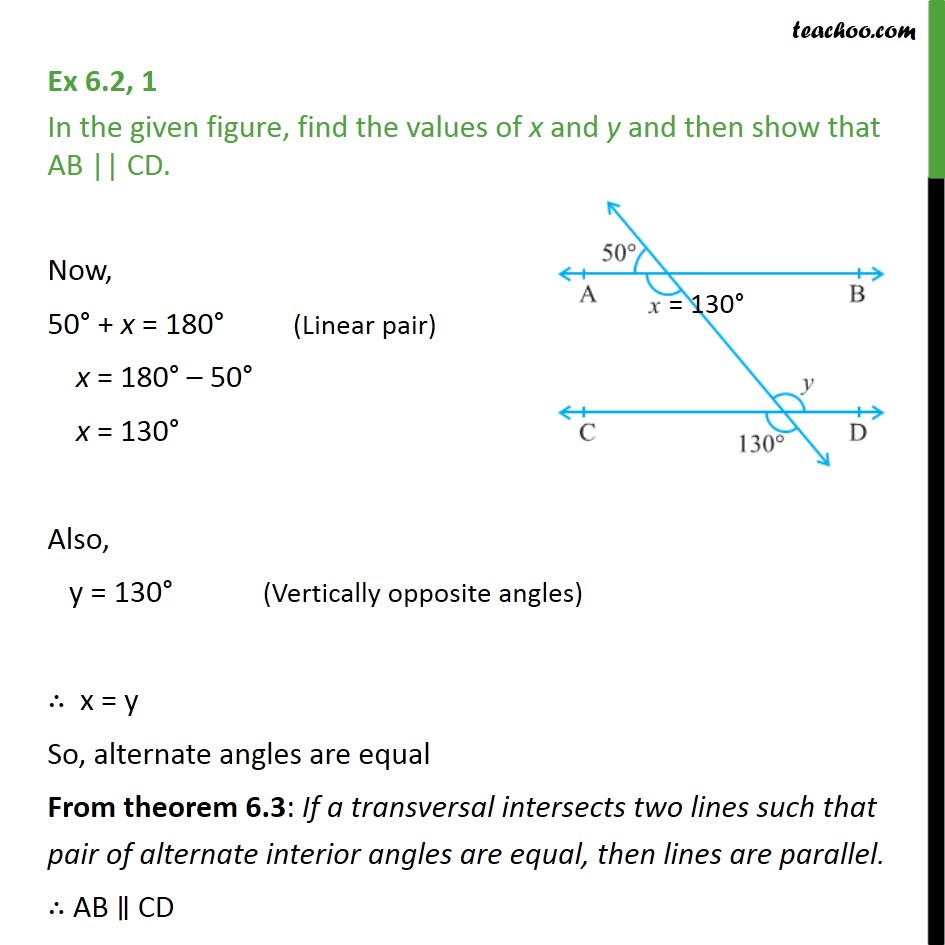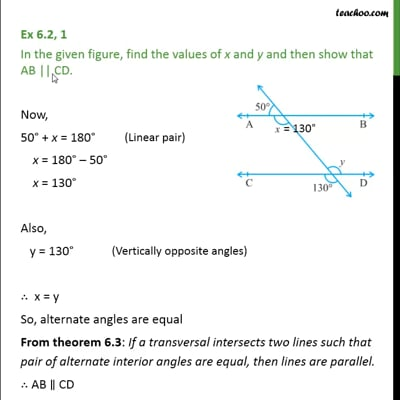Ex 6.2

Chapter 6 Class 9 Lines and Angles (Term 1)
Serial order wiseThis video is only available for Teachoo black users

### Transcript

Ex 6.2, 1 In the given figure, find the values of x and y and then show that AB || CD. Now, 50 + x = 180 x = 180 50 x = 130 Also, y = 130 x = y So, alternate angles are equal From theorem 6.3: If a transversal intersects two lines such that pair of alternate interior angles are equal, then lines are parallel. AB CD